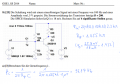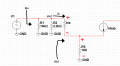# Cut off frequency at a emitter circuit

#### Tobias Hildebrandt

Joined Jan 1, 2016
44Hello,

This is the information provided on top of the page: A sine shaped signal with a frequency of 100 Hz and an amplitude of 1.5V is put in the circuit. The current amplification of the transistor is B = 200. A SPICE simulation proivdes for Ic(Q1) a current of 18 mA. Provide your calculations down to four significant figures.

a) Calculate the cutoff frequency (fc).

This is my question. Where does the 20.1 kOhm coming from? He calculate "little re" as 25.8 mV / 18 mA = 1.433 Ohm. Now, this is what I did next, I added "little re" to R4 and multiplied it by 200 ( (1.433 Ohm + 100 Ohm) * 200) and got as a result 20286.6 Ohm, rounded this is 20.3 kOhm.

So, what is wrong with my approach?

Tobias

#### Jony130

Joined Feb 17, 2009
5,250
The input impedance for this amp is
Rin = R1||R2||RinT where

RinT = (β+1)*(R4 + r'e) and because r'e<<R4 we can use ≈ (β+1) * R4 = 20.1kΩ
Why (β+1) ??
Because the emitter current is Ie = Ic + Ib = β*Ic + Ib = (β + 1)*Ib

•Tobias Hildebrandt

#### MrAl

Joined Jun 17, 2014
8,540
Hi,

I thought we talked about this before. The more correct way is (B+1)*R4 not B*R4 but sometimes B*R4 is acceptable. If it is shown on the text then they want you to use (B+1)*R4 this time.

Little re is the internal transistor emitter resistance, which in this case is very small (about 0.15 percent of external R4) so it can be neglected as Jony said.
Little re is more important sometimes but not for this circuit. The idea probably is that you should calculate the approximate little re (as they show) and determine for yourself if it is significant or not. Little re times (B+1) is around 300 ohms, while R4*(B+1) is 20.1k, so the combined total is 20.4k which isnt that different than 20.1k so it only makes a little difference.

•Tobias Hildebrandt

#### Tobias Hildebrandt

Joined Jan 1, 2016
44
Thanks to both of you! Yes, we went over this before, but I got puzzled when I saw the "little re" calculation in his solution.

So, I can ignore "little re" when << R4. Good!

#### BramLabs

Joined Nov 21, 2013
98
If i applied small signal analysis :Rin = R1//R2//RinT

And applied KVL for finding RinT =
V at hie + V at R4 = VinT
hie*ib + (hfe*ib+ib) * R4 = VinT
because hfe = B, then :
hie*ib + (B+1) * ib* R4 = VinT
(B+1) * ib * (R4+hie) = VinT
Then : VinT / ib = RinT = (B+1)*(R4+hie)
And if hie at least 10 times less than R4, we can say :
RinT = (B+1)*R4

CMIIW (Correct Me If I'm Wrong)

Last edited: